Homework Help Question & Answers

# As Details A 30 kilogram mass is attached to a spring. If the frequency of mls...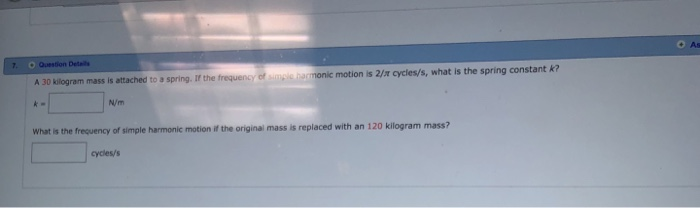As Details A 30 kilogram mass is attached to a spring. If the frequency of mls namonic motion is 2/m cycles/s, what is the spring constant k N/m What is the frequency of simple harmonic motion if the original mass is replaced with an 120 kilogram mass? cycles/s

#### Homework Answers

Answer #1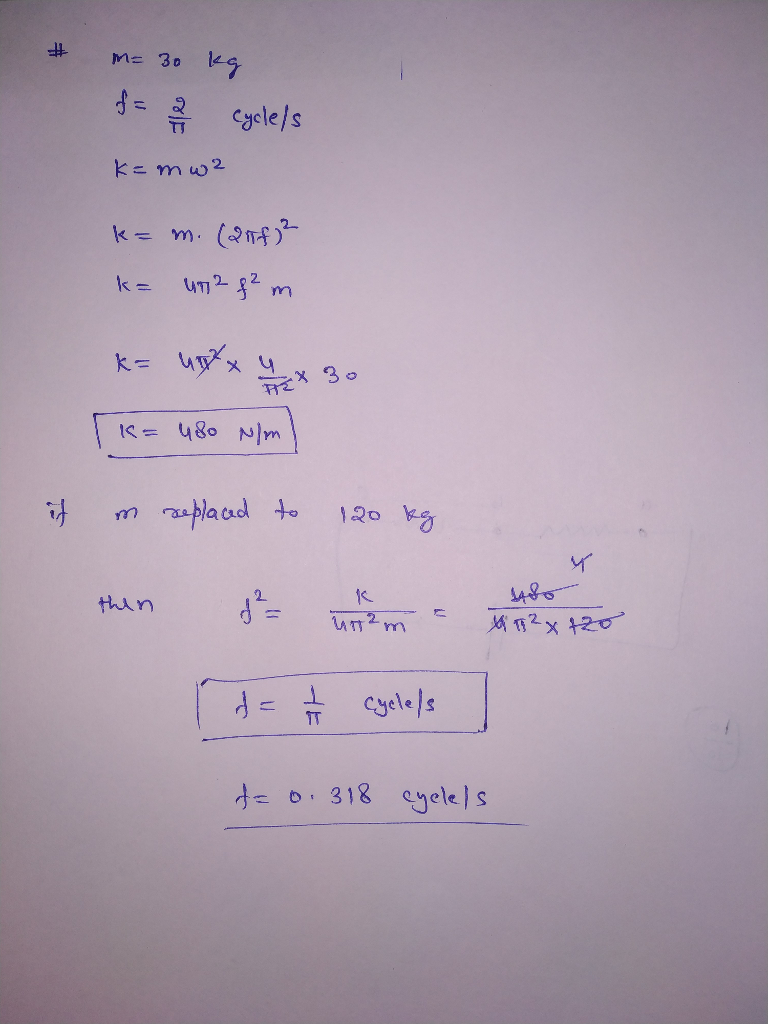Know the answer?
Your Answer:

#### Post as a guest

Your Name:

What's your source?

#### Earn Coin

Coins can be redeemed for fabulous gifts.

Not the answer you're looking for? Ask your own homework help question. Our experts will answer your question WITHIN MINUTES for Free.
Similar Homework Help Questions
• ### A mass of 0.160kg is attached to a spring of spring constant 41.0 N/m if the...

A mass of 0.160kg is attached to a spring of spring constant 41.0 N/m if the mass executes simple harmonic motion, what will be the period (T) of its motion ?

• ### QUESTION 10 When a 200 g mass attached to a horizontal spring (k= 25 N/m) is...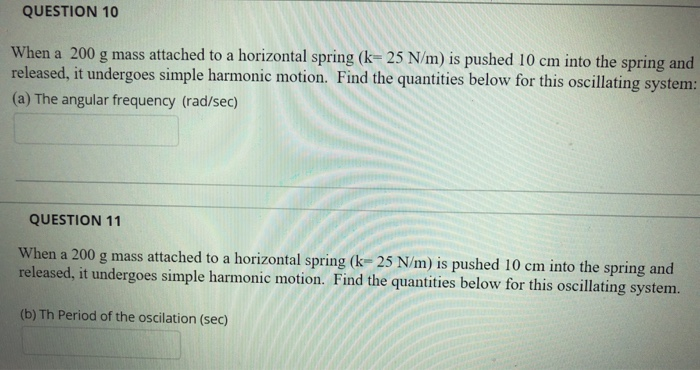QUESTION 10 When a 200 g mass attached to a horizontal spring (k= 25 N/m) is pushed 10 cm into the spring and released, it undergoes simple harmonic motion. Find the quantities below for this oscillating system: (a) The angular frequency (rad/sec) QUESTION 11 When a 200 g mass attached to a horizontal spring (k-25 N/m) is pushed 10 cm into the spring and released, it undergoes simple harmonic motion. Find the quantities below for this oscillating system. (b) Th...

• ### An object with mass 3.5 kg is attached to a spring with spring stiffness constant k...

An object with mass 3.5 kg is attached to a spring with spring stiffness constant k = 250 N/m and is executing simple harmonic motion. When the object is 0.020 m from its equilibrium position, it is moving with a speed of 0.55 m/s. (a) Calculate the amplitude of the motion. _______________________________ m (b) Calculate the maximum velocity attained by the object. [Hint: Use conservation of energy.] _______________________________ m/s

• ### A block of mass 1.20 kg is attached to a horizontal spring that has force constant...

A block of mass 1.20 kg is attached to a horizontal spring that has force constant k = 300 N/m. The block moves on a horizontal frictionless surface. The maximum speed of the block during its motion is 5 m/s. What is the amplitude A of the simple harmonic motion of the block?

• ### 2. A block of unknown mass is attached to a spring with a spring constant of...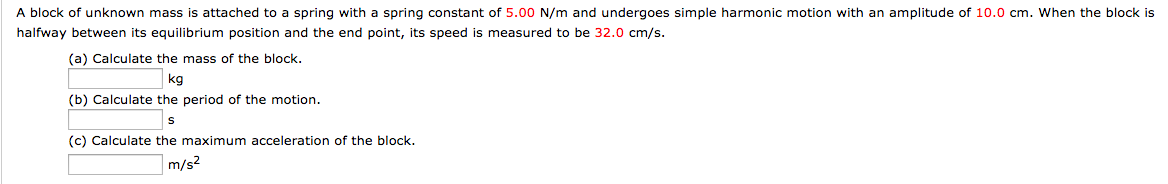2. A block of unknown mass is attached to a spring with a spring constant of 5.00 N/m and undergoes simple harmonic motion with an amplitude of 10.0 cm. When the block is halfway between its equilibrium position and the end point, its speed is measured to be 32.0 cm/s (a) Calculate the mass of the block (b) Calculate the period of the motion (c) Calculate the maximum acceleration of the block. kg m/s

• ### Problem 2. A simple harmonic oscillator consists of a mass m attached to a spring with...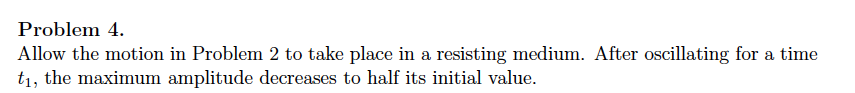Problem 2. A simple harmonic oscillator consists of a mass m attached to a spring with spring constant k. The mass is displaced a distance a and released from rest. v0 is the nature frequency. Problem 4 Allow the motion in Problem 2 to take place in a resisting medium. After oscillating for a time t1, the maximum amplitude decreases to half its initial value

• ### A 0.8 kg mass attached to a vertical spring undergoes simple harmonic motion with a frequency...

A 0.8 kg mass attached to a vertical spring undergoes simple harmonic motion with a frequency of 0.5 Hz. a) What is the period of the motion and the spring constant? b) If the amplitude of oscillation is 10 cm and the mass starts at its lowest point at time zero, write the equation describing the displacement of the mass as a function of time and find the position of the mass at times 1, 2, 1.5 s, and 1.25...

• ### A horizontal mass-spring system consists of a 2 kg mass moving on a frictionless surface attached...

A horizontal mass-spring system consists of a 2 kg mass moving on a frictionless surface attached to a spring. The other end of the spring is attached to a wall. The mass is pulled and released. The resultant simple harmonic motion has a period of 5 s and it is observed that the maximum velocity of the mass is 0.3 m/s. a) Calculate the spring constant of the spring. (b) Calculate the amplitude of the motion. Sometime later, when the...

• ### When a 200 g mass attached to a horizontal spring (k= 25 N/m) is pushed 10...

When a 200 g mass attached to a horizontal spring (k= 25 N/m) is pushed 10 cm into the spring and released, it undergoes simple harmonic motion. Find the quantities below for this oscillating system: (a) The angular frequency (rad/sec) b) Th Period of the oscilation (sec) c) The frequency (Hz) d) The maximum speed (m/s) (e) Maximum acceleration (m/s2)

• ### A mass of 0.200kg is attached to a spring of length 0.500m and is placed on...

A mass of 0.200kg is attached to a spring of length 0.500m and is placed on a horizontal frictionless surface. The mass is then pulled so that the spring now stretches to a total length of 0.550m. The mass is then given an initial speed of 5.00 m/s and the mass undergoes simple harmonic motion with a period of 0.200s. Find the spring constant (k) of the spring. Find the amplitude oscillation of the mass. Find the speed of the...